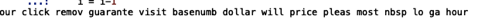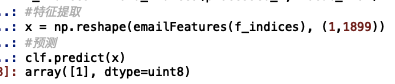2017-09-05 14:25:48 dangyalingengjia 阅读数 2036
• ###### AI时代，机器学习该如何入门？

机器学习入门视频教程，该课程会告诉大家如何入门机器学习，掌握机器学习必要的基础知识，了解机器学习路径。对于机器学习，很多人的观点是：机器学习技术是今后所有技术人员都绕不过的一个门槛。 那么，普通程序员该学习机器学作为一名对机器学习心有向往的程序员，我该以什么样的姿势开始呢？不妨看看该课程。

7197 人正在学习 去看看 CSDN讲师

1，首先提取分类特征

2，根据特征值计算新邮件是垃圾邮件的可能性。P(是垃圾邮件 | 含特征词w1,…wn) = P(含特征词w1 | 是垃圾邮件)*…P(wn | 是垃圾邮件) * P(是垃圾邮件) / (P(w1) * … P(wn))

3，解决误杀和漏杀的问题。

2017-09-06 11:15:01 gyh_420 阅读数 352
• ###### AI时代，机器学习该如何入门？

机器学习入门视频教程，该课程会告诉大家如何入门机器学习，掌握机器学习必要的基础知识，了解机器学习路径。对于机器学习，很多人的观点是：机器学习技术是今后所有技术人员都绕不过的一个门槛。 那么，普通程序员该学习机器学作为一名对机器学习心有向往的程序员，我该以什么样的姿势开始呢？不妨看看该课程。

7197 人正在学习 去看看 CSDN讲师http://openclassroom.stanford.edu/MainFolder/courses/MachineLearning/exercises/ex6materials/ex6DataPrepared.zip

http://openclassroom.stanford.edu/MainFolder/DocumentPage.php?course=MachineLearning&doc=exercises/ex6/ex6.htmlm代表有m个特征值（x）,φj表示第j个特征向量xj的概率,^表示and的意思，所以我们在使用的时候就是算出xj的个数，除以分母即是φj|y=1和φj|y=0的值。m代表有m个文本，本试验中有700的文本用例，k代表的是对应的特征词，ni表示第i个文本中有ni个特征词，V代表的是特征数量。% train.m
% Exercise 6: Naive Bayes text classifier

clear all; close all; clc

% store the number of training examples
numTrainDocs = 700;

% store the dictionary size
numTokens = 2500;

spmatrix = sparse(M(:,1), M(:,2), M(:,3), numTrainDocs, numTokens);
train_matrix = full(spmatrix);

% train_matrix now contains information about the words within the emails
% the i-th row of train_matrix represents the i-th training email
% for a particular email, the entry in the j-th column tells
% you how many times the j-th dictionary word appears in that email

% the i-th entry of train_labels now indicates whether document i is spam

% Find the indices for the spam and nonspam labels
spam_indices = find(train_labels);
nonspam_indices = find(train_labels == 0);

% Calculate probability of spam
prob_spam = length(spam_indices) / numTrainDocs;

% Sum the number of words in each email by summing along each row of
% train_matrix
email_lengths = sum(train_matrix, 2);%得到每个邮件中的特征词的个数,ni个
% Now find the total word counts of all the spam emails and nonspam emails
spam_wc = sum(email_lengths(spam_indices));%代表∑1{y(i)=1}ni
nonspam_wc = sum(email_lengths(nonspam_indices));%代表∑1{y(i)=0}ni

% Calculate the probability of the tokens in spam emails
%对应于∑∑1{xj^i=K and y(i)=1}+1
prob_tokens_spam = (sum(train_matrix(spam_indices, :)) + 1) ./ ...
(spam_wc + numTokens);
% Now the k-th entry of prob_tokens_spam represents phi_(k|y=1)

% Calculate the probability of the tokens in non-spam emails
prob_tokens_nonspam = (sum(train_matrix(nonspam_indices, :)) + 1)./ ...
(nonspam_wc + numTokens);
% Now the k-th entry of prob_tokens_nonspam represents phi_(k|y=0)


% test.m
% Exercise 6: Naive Bayes text classifier

% read the test matrix in the same way we read the training matrix
spmatrix = sparse(N(:,1), N(:,2), N(:,3));
test_matrix = full(spmatrix);

% Store the number of test documents and the size of the dictionary
numTestDocs = size(test_matrix, 1);
numTokens = size(test_matrix, 2);

% The output vector is a vector that will store the spam/nonspam prediction
% for the documents in our test set.
output = zeros(numTestDocs, 1);

% Calculate log p(x|y=1) + log p(y=1)
% and log p(x|y=0) + log p(y=0)
% for every document
% make your prediction based on what value is higher
% (note that this is a vectorized implementation and there are other
%  ways to calculate the prediction)
log_a = test_matrix*(log(prob_tokens_spam))' + log(prob_spam);
log_b = test_matrix*(log(prob_tokens_nonspam))'+ log(1 - prob_spam);
output = log_a > log_b;

% Read the correct labels of the test set

% Compute the error on the test set
% A document is misclassified if it's predicted label is different from
% the actual label, so count the number of 1's from an exclusive "or"
numdocs_wrong = sum(xor(output, test_labels))

%Print out error statistics on the test set
fraction_wrong = numdocs_wrong/numTestDocs2018-08-01 19:59:39 imHery 阅读数 302
• ###### AI时代，机器学习该如何入门？

机器学习入门视频教程，该课程会告诉大家如何入门机器学习，掌握机器学习必要的基础知识，了解机器学习路径。对于机器学习，很多人的观点是：机器学习技术是今后所有技术人员都绕不过的一个门槛。 那么，普通程序员该学习机器学作为一名对机器学习心有向往的程序员，我该以什么样的姿势开始呢？不妨看看该课程。

7197 人正在学习 去看看 CSDN讲师

def setOfWords2Vec(vocabList, inputSet):#输入：词表向量+待测文本向量
returnVec = *len(vocabList)
for eachWord in inputSet:
if eachWord in vocabList:
returnVec[vocabList.index(eachWord)] = 1
else: print "the word: %s is not in my Vocabulary!" % eachWord
return returnVec#返回词表0/1向量，反映那些词汇在文档中是否出现

def bagOfWords2VecMN(vocabList, inputSet):#反映词表中单词在文档中出现次数
returnVec = *len(vocabList)
for word in inputSet:
if word in vocabList:
returnVec[vocabList.index(word)] += 1
return returnVec#返回数字向量

def textParse(bigString):    #input is big string, #output is word list
import re
listOfTokens = re.split(r'\W*', bigString)
return [tok.lower() for tok in listOfTokens if len(tok) > 2] 

def spamTest():
docList=[]
classList = []
fullText =[]

for i in range(1,26):
#读取垃圾邮件
docList.append(wordList)  #list-list
fullText.extend(wordList) #list
classList.append(1)
#读取正常邮件
docList.append(wordList)
fullText.extend(wordList)
classList.append(0)

vocabList = createVocabList(docList)#词表向量

trainingSet = range(50)#50个号码
testSet=[]             #创建测试集
for i in range(10):    #随机抽取10个号码
randIndex = int(random.uniform(0,len(trainingSet)))
testSet.append(trainingSet[randIndex])
del(trainingSet[randIndex])
trainMat=[]
trainClasses = []
for docIndex in trainingSet:#range(50)剩下的40个号码
trainMat.append(bagOfWords2VecMN(vocabList, docList[docIndex]))
trainClasses.append(classList[docIndex])
p0V,p1V,pSpam = trainNB0(array(trainMat),array(trainClasses))

errorCount = 0
for docIndex in testSet:    #10个随机号码
wordVector = bagOfWords2VecMN(vocabList, docList[docIndex])
if classifyNB(array(wordVector),p0V,p1V,pSpam) != classList[docIndex]:
errorCount += 1
print "classification error\n",docList[docIndex]
print "classify value:",classifyNB(array(wordVector),p0V,p1V,pSpam)
print "real value:",classList[docIndex]
print "***********************************************************"
print 'the error rate is: ',float(errorCount)/len(testSet)
#return vocabList,fullText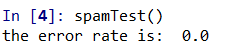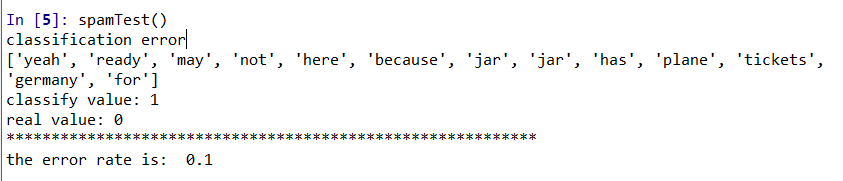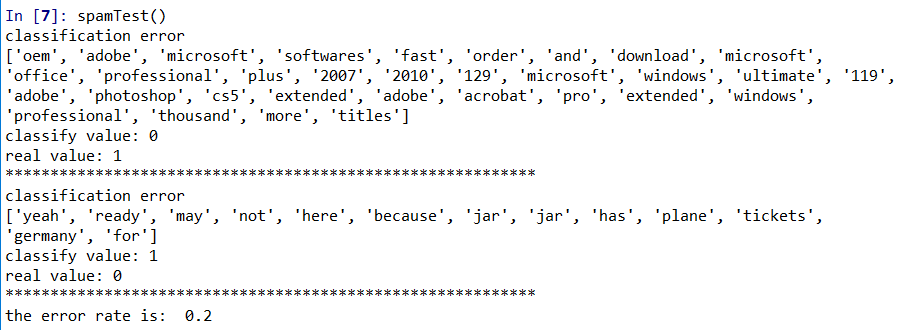2019-03-30 18:38:27 qq_29317617 阅读数 338
• ###### AI时代，机器学习该如何入门？

机器学习入门视频教程，该课程会告诉大家如何入门机器学习，掌握机器学习必要的基础知识，了解机器学习路径。对于机器学习，很多人的观点是：机器学习技术是今后所有技术人员都绕不过的一个门槛。 那么，普通程序员该学习机器学作为一名对机器学习心有向往的程序员，我该以什么样的姿势开始呢？不妨看看该课程。

7197 人正在学习 去看看 CSDN讲师

## 我们的任务

### 第 1.1 步：了解我们的数据集

** 下面是该数据的预览：**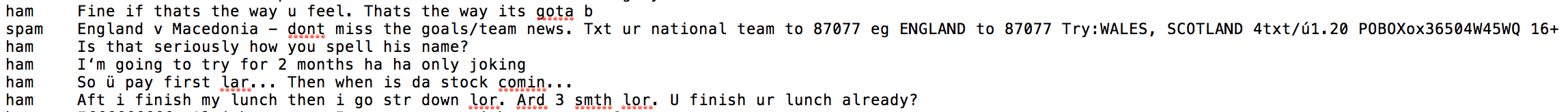** 说明：**

• 使用 read_table 方法可以将数据集导入 pandas 数据帧。因为这是一个用制表符分隔的数据集，因此我们将使用“\t”作为“sep”参数的值，表示这种分隔格式。
• 此外，通过为 read_table() 的“names”参数指定列表 [‘label’, ‘sms_message’]，重命名列。
• 用新的列名输出数据帧的前五个值。
'''
Solution
'''
import pandas as pd
# Dataset from - https://archive.ics.uci.edu/ml/datasets/SMS+Spam+Collection
df = pd.read_table('smsspamcollection/SMSSpamCollection', sep='\t', names=['label', 'sms_message'])

# Output printing out first 5 columns

label sms_message
0 ham Go until jurong point, crazy.. Available only ...
1 ham Ok lar... Joking wif u oni...
2 spam Free entry in 2 a wkly comp to win FA Cup fina...
3 ham U dun say so early hor... U c already then say...
4 ham Nah I don't think he goes to usf, he lives aro...

### 第 1.2 步：数据预处理

• 使用映射方法将“标签”列中的值转换为数字值，如下所示：
{‘ham’:0, ‘spam’:1} 这样会将“ham”值映射为 0，将“spam”值映射为 1。
• 此外，为了知道我们正在处理的数据集有多大，使用“shape”输出行数和列数
'''
Solution
'''
print(df.shape)
df['label'] = df.label.map({'ham':0, 'spam':1})

(5572, 2)

df['label'].head()

0    0
1    0
2    1
3    0
4    0
Name: label, dtype: int64


### 第 2.1 步：Bag of words

['Hello, how are you!', 'Win money, win from home.', 'Call me now', 'Hello, Call you tomorrow?']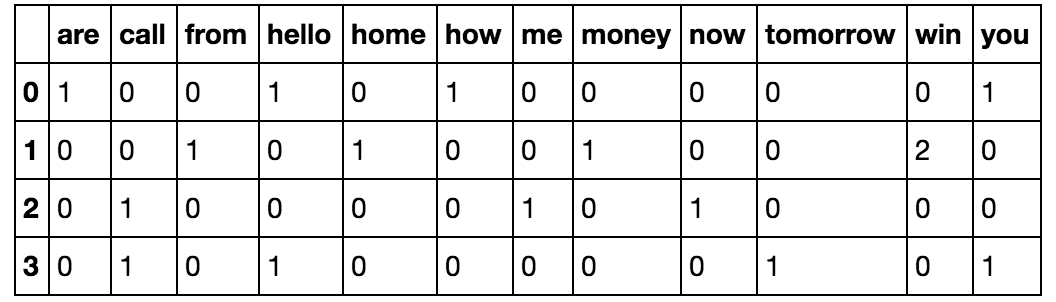count vectorizer 方法，该方法的作用如下所示：

• 它会令牌化字符串（将字符串划分为单个单词）并为每个令牌设定一个整型 ID。
• 它会计算每个令牌的出现次数。

** 请注意：**

• CountVectorizer 方法会自动将所有令牌化单词转换为小写形式，避免区分“He”和“he”等单词。为此，它会使用参数 lowercase，该参数默认设为 True
• 它还会忽略所有标点符号，避免区分后面有标点的单词（例如“hello!”）和前后没有标点的同一单词（例如“hello”）。为此，它会使用参数 token_pattern，该参数使用默认正则表达式选择具有 2 个或多个字母数字字符的令牌。
• 要注意的第三个参数是 stop_words。停用词是指某个语言中最常用的字词，包括“am”、“an”、“and”、“the”等。 通过将此参数值设为 english，CountVectorizer 将自动忽略（输入文本中）出现在 scikit-learn 中的内置英语停用词列表中的所有单词。这非常有用，因为当我们尝试查找表明是垃圾内容的某些单词时，停用词会使我们的结论出现偏差。

### 第 2.2 步：从头实现 Bag of Words

** 第 1 步：将所有字符串转换成小写形式。**

documents = ['Hello, how are you!',
'Win money, win from home.',
'Call me now.',
'Hello, Call hello you tomorrow?']


** 说明：**

• 将文档集合中的所有字符串转换成小写形式。将它们保存到叫做“lower_case_documents”的列表中。你可以使用 lower() 方法在 python 中将字符串转换成小写形式。
'''
Solution:
'''
documents = ['Hello, how are you!',
'Win money, win from home.',
'Call me now.',
'Hello, Call hello you tomorrow?']

lower_case_documents = []
for i in documents:
i = i.lower()
lower_case_documents.append(i)
print(lower_case_documents)

['hello, how are you!', 'win money, win from home.', 'call me now.', 'hello, call hello you tomorrow?']


** 第 2 步：删除所有标点符号 **

'''
Solution:
'''
sans_punctuation_documents = []
import string

for i in lower_case_documents:
# TODO
i = i.translate(str.maketrans('', '', string.punctuation))
sans_punctuation_documents.append(i)

print(sans_punctuation_documents)

['hello how are you', 'win money win from home', 'call me now', 'hello call hello you tomorrow']


** 第 3 步：令牌化 **

'''
Solution:
'''
preprocessed_documents = []
for i in sans_punctuation_documents:
i = i.split(' ')
preprocessed_documents.append(i)
print(preprocessed_documents)

[['hello', 'how', 'are', 'you'], ['win', 'money', 'win', 'from', 'home'], ['call', 'me', 'now'], ['hello', 'call', 'hello', 'you', 'tomorrow']]


** 第 4 步：计算频率 **

Counter 会数出列表中每项的出现次数，并返回一个字典，键是被数的项目，相应的值是该项目在列表中的计数。

'''
Solution
'''
frequency_list = []
import pprint
from collections import Counter

for i in preprocessed_documents:
#TODO
frequency_list.append(Counter(i))

pprint.pprint(frequency_list)

[Counter({'hello': 1, 'how': 1, 'are': 1, 'you': 1}),
Counter({'win': 2, 'money': 1, 'from': 1, 'home': 1}),
Counter({'call': 1, 'me': 1, 'now': 1}),
Counter({'hello': 2, 'call': 1, 'you': 1, 'tomorrow': 1})]


### 第 2.3 步：在 scikit-learn 中实现 Bag of Words

'''
Here we will look to create a frequency matrix on a smaller document set to make sure we understand how the
document-term matrix generation happens. We have created a sample document set 'documents'.
'''
documents = ['Hello, how are you!',
'Win money, win from home.',
'Call me now.',
'Hello, Call hello you tomorrow?']


'''
Solution
'''
from sklearn.feature_extraction.text import CountVectorizer
count_vector = CountVectorizer()


** 使用 CountVectorizer() 预处理数据 **

• lowercase = True

lowercase 参数的默认值为 True，它会将所有文本都转换为小写形式。

• token_pattern = (?u)\\b\\w\\w+\\b

token_pattern 参数具有默认正则表达式值 (?u)\\b\\w\\w+\\b，它会忽略所有标点符号并将它们当做分隔符，并将长度大于等于 2 的字母数字字符串当做单个令牌或单词。

• stop_words

stop_words 参数如果设为 english，将从文档集合中删除与 scikit-learn 中定义的英语停用词列表匹配的所有单词。考虑到我们的数据集规模不大，并且我们处理的是信息，并不是电子邮件这样的更庞大文本来源，因此我们将不设置此参数值。

'''
Practice node:
Print the 'count_vector' object which is an instance of 'CountVectorizer()'
'''
print(count_vector)

CountVectorizer(analyzer='word', binary=False, decode_error='strict',
dtype=<class 'numpy.int64'>, encoding='utf-8', input='content',
lowercase=True, max_df=1.0, max_features=None, min_df=1,
ngram_range=(1, 1), preprocessor=None, stop_words=None,
strip_accents=None, token_pattern='(?u)\\b\\w\\w+\\b',
tokenizer=None, vocabulary=None)


'''
Solution:
'''
count_vector.fit(documents)
count_vector.get_feature_names()

['are',
'call',
'from',
'hello',
'home',
'how',
'me',
'money',
'now',
'tomorrow',
'win',
'you']


get_feature_names() 方法会返回此数据集的特征名称，即组成 ‘documents’ 词汇表的单词集合。

**

'''
Solution
'''
doc_array = count_vector.transform(documents).toarray()
doc_array

array([[1, 0, 0, 1, 0, 1, 0, 0, 0, 0, 0, 1],
[0, 0, 1, 0, 1, 0, 0, 1, 0, 0, 2, 0],
[0, 1, 0, 0, 0, 0, 1, 0, 1, 0, 0, 0],
[0, 1, 0, 2, 0, 0, 0, 0, 0, 1, 0, 1]])


'''
Solution
'''
frequency_matrix = pd.DataFrame(doc_array, columns = count_vector.get_feature_names())
frequency_matrix

are call from hello home how me money now tomorrow win you
0 1 0 0 1 0 1 0 0 0 0 0 1
1 0 0 1 0 1 0 0 1 0 0 2 0
2 0 1 0 0 0 0 1 0 1 0 0 0
3 0 1 0 2 0 0 0 0 0 1 0 1

### 第 3.1 步：训练集和测试集

• X_train 是 ‘sms_message’ 列的训练数据。
• y_train 是 ‘label’ 列的训练数据
• X_test 是 ‘sms_message’ 列的测试数据。
• y_test 是 ‘label’ 列的测试数据。
输出每个训练数据和测试数据的行数。
'''
Solution

NOTE: sklearn.cross_validation will be deprecated soon to sklearn.model_selection
'''
# split into training and testing sets
# USE from sklearn.model_selection import train_test_split to avoid seeing deprecation warning.
from sklearn.cross_validation import train_test_split

X_train, X_test, y_train, y_test = train_test_split(df['sms_message'],
df['label'],
random_state=1)

print('Number of rows in the total set: {}'.format(df.shape))
print('Number of rows in the training set: {}'.format(X_train.shape))
print('Number of rows in the test set: {}'.format(X_test.shape))

Number of rows in the total set: 5572
Number of rows in the training set: 4179
Number of rows in the test set: 1393


### 第 3.2 步：对数据集应用 Bag of Words 流程。

• 首先，我们需要对 CountVectorizer()拟合训练数据 (X_train) 并返回矩阵。
• 其次，我们需要转换测试数据 (X_test) 以返回矩阵。

X_test 是 ‘sms_message’ 列的测试数据，我们将使用该数据（转换为矩阵后）进行预测。然后在后面的步骤中将这些预测与 y_test 进行比较。

'''
[Practice Node]

The code for this segment is in 2 parts. Firstly, we are learning a vocabulary dictionary for the training data
and then transforming the data into a document-term matrix; secondly, for the testing data we are only
transforming the data into a document-term matrix.

This is similar to the process we followed in Step 2.3

We will provide the transformed data to students in the variables 'training_data' and 'testing_data'.
'''

"\n[Practice Node]\n\nThe code for this segment is in 2 parts. Firstly, we are learning a vocabulary dictionary for the training data \nand then transforming the data into a document-term matrix; secondly, for the testing data we are only \ntransforming the data into a document-term matrix.\n\nThis is similar to the process we followed in Step 2.3\n\nWe will provide the transformed data to students in the variables 'training_data' and 'testing_data'.\n"

'''
Solution
'''
# Instantiate the CountVectorizer method
count_vector = CountVectorizer()

# Fit the training data and then return the matrix
training_data = count_vector.fit_transform(X_train)

# Transform testing data and return the matrix. Note we are not fitting the testing data into the CountVectorizer()
testing_data = count_vector.transform(X_test)


### 第 4.1 步：从头实现贝叶斯定理

P(D) 是某人患有糖尿病的概率。值为 0.01，换句话说，普通人群中有 1% 的人患有糖尿病（免责声明：这些值只是假设，并非任何医学研究的结论）。

P(Pos)：是获得阳性测试结果的概率。

P(Neg)：是获得阴性测试结果的概率。

P(Pos|D)：是本身有糖尿病并且获得阳性测试结果的概率，值为 0.9，换句话说，该测试在 90% 的情况下是正确的。亦称为敏感性或真正例率。

P(Neg|~D)：是本身没有糖尿病并且获得阴性测试结果的概率，值也为 0.9 ，因此在 90% 的情况下是正确的。亦称为特异性或真负例率。• P(A)：A 独立发生的先验概率。在我们的示例中为 P(D)，该值已经提供给我们了 。

• P(B)：B 独立发生的先验概率。在我们的示例中为 P(Pos)

• P(A|B)：在给定 B 的情况下 A 发生的后验概率，在我们的示例中为 P(D|Pos)，即某人的测试结果为阳性时患有糖尿病的概率。这是我们要计算的值。

• P(B|A)：在给定 A 的情况下 B 可能发生的概率。在我们的示例中为 P(Pos|D)，该值已经提供给我们了 。

P(D|Pos) = P(D) * P(Pos|D) / P(Pos)

P(Pos) = [P(D) * Sensitivity] + [P(~D) * (1-Specificity))]

'''
Instructions:
Calculate probability of getting a positive test result, P(Pos)
'''

'''
Solution (skeleton code will be provided)
'''
# P(D)
p_diabetes = 0.01

# P(~D)
p_no_diabetes = 0.99

# Sensitivity or P(Pos|D)
p_pos_diabetes = 0.9

# Specificity or P(Neg|~D)
p_neg_no_diabetes = 0.9

# P(Pos)
p_pos = p_pos_diabetes*p_diabetes + (1 - p_neg_no_diabetes)*p_no_diabetes
print('The probability of getting a positive test result P(Pos) is: {}'.format(p_pos))

The probability of getting a positive test result P(Pos) is: 0.10799999999999998


** 我们可以利用所有这些信息计算后验概率，如下所示：**

P(D|Pos) = (P(D) * Sensitivity)) / P(Pos)

P(~D|Pos) = (P(~D) * (1-Specificity)) / P(Pos)

'''
Instructions:
Compute the probability of an individual having diabetes, given that, that individual got a positive test result.
In other words, compute P(D|Pos).

The formula is: P(D|Pos) = (P(D) * P(Pos|D) / P(Pos)
'''

'''
Solution
'''
# P(D|Pos)
p_diabetes_pos = (p_pos_diabetes*p_diabetes)/p_pos
print('Probability of an individual having diabetes, given that that individual got a positive test result is:\
',format(p_diabetes_pos))

Probability of an individual having diabetes, given that that individual got a positive test result is: 0.08333333333333336

'''
Instructions:
Compute the probability of an individual not having diabetes, given that, that individual got a positive test result.
In other words, compute P(~D|Pos).

The formula is: P(~D|Pos) = P(~D) * P(Pos|~D) / P(Pos)

Note that P(Pos|~D) can be computed as 1 - P(Neg|~D).

Therefore:
P(Pos|~D) = p_pos_no_diabetes = 1 - 0.9 = 0.1
'''

'''
Solution
'''
# P(Pos|~D)
p_pos_no_diabetes = 0.1

# P(~D|Pos)
p_no_diabetes_pos = (p_pos_no_diabetes*p_no_diabetes) / p_pos
print('Probability of an individual not having diabetes, given that that individual got a positive test result is:'\
,p_no_diabetes_pos)

Probability of an individual not having diabetes, given that that individual got a positive test result is: 0.9166666666666669


** “朴素贝叶斯”中的“朴素”一词是什么意思？**

### 第 4.2 步：从头实现朴素贝叶斯

• Jill Stein 提到“自由”的概率：0.1 ---------> P(F|J)

• Jill Stein 提到“移民”的概率：0.1 -----> P(I|J)

• Jill Stein 提到“环境”的概率：0.8 -----> P(E|J)

• Gary Johnson 提到“自由”的概率：0.7 -------> P(F|G)

• Gary Johnson 提到“移民”的概率：0.2 —> P(I|G)

• Gary Johnson 提到“环境”的概率：0.1 —> P(E|G)• P(J|F,I)：Jill Stein 提到“自由”和“移民”的概率。

• P(G|F,I)：Gary Johnson 提到“自由”和“移民”的概率。

'''
Instructions: Compute the probability of the words 'freedom' and 'immigration' being said in a speech, or
P(F,I).

The first step is multiplying the probabilities of Jill Stein giving a speech with her individual
probabilities of saying the words 'freedom' and 'immigration'. Store this in a variable called p_j_text

The second step is multiplying the probabilities of Gary Johnson giving a speech with his individual
probabilities of saying the words 'freedom' and 'immigration'. Store this in a variable called p_g_text

The third step is to add both of these probabilities and you will get P(F,I).
'''

'''
Solution: Step 1
'''
# P(J)
p_j = 0.5

# P(F/J)
p_j_f = 0.1

# P(I/J)
p_j_i = 0.1

p_j_text = p_j * p_j_f * p_j_i
print(p_j_text)

0.005000000000000001

'''
Solution: Step 2
'''
# P(G)
p_g = 0.5

# P(F/G)
p_g_f = 0.7

# P(I/G)
p_g_i = 0.2

p_g_text = p_g * p_g_f * p_g_i
print(p_g_text)

0.06999999999999999

'''
Solution: Step 3: Compute P(F,I) and store in p_f_i
'''
p_f_i = p_j_text + p_g_text
print('Probability of words freedom and immigration being said are: ', format(p_f_i))

Probability of words freedom and immigration being said are:  0.075


'''
Instructions:
Compute P(J|F,I) using the formula P(J|F,I) = (P(J) * P(F|J) * P(I|J)) / P(F,I) and store it in a variable p_j_fi
'''

'''
Solution
'''
p_j_fi = p_j_text / p_f_i
print('The probability of Jill Stein saying the words Freedom and Immigration: ', format(p_j_fi))

The probability of Jill Stein saying the words Freedom and Immigration:  0.06666666666666668

'''
Instructions:
Compute P(G|F,I) using the formula P(G|F,I) = (P(G) * P(F|G) * P(I|G)) / P(F,I) and store it in a variable p_g_fi
'''

'''
Solution
'''
p_g_fi = p_g_text / p_f_i
print('The probability of Gary Johnson saying the words Freedom and Immigration: ', format(p_g_fi))

The probability of Gary Johnson saying the words Freedom and Immigration:  0.9333333333333332


### 第 5 步：使用 scikit-learn 实现朴素贝叶斯

'''
Instructions:

We have loaded the training data into the variable 'training_data' and the testing data into the
variable 'testing_data'.

Import the MultinomialNB classifier and fit the training data into the classifier using fit(). Name your classifier
'naive_bayes'. You will be training the classifier using 'training_data' and y_train' from our split earlier.
'''

'''
Solution
'''
from sklearn.naive_bayes import MultinomialNB
naive_bayes = MultinomialNB()
naive_bayes.fit(training_data, y_train)

MultinomialNB(alpha=1.0, class_prior=None, fit_prior=True)

'''
Instructions:
Now that our algorithm has been trained using the training data set we can now make some predictions on the test data
stored in 'testing_data' using predict(). Save your predictions into the 'predictions' variable.
'''

'''
Solution
'''
predictions = naive_bayes.predict(testing_data)


### 第 6 步：评估模型

** 准确率 **衡量的是分类器做出正确预测的概率，即正确预测的数量与预测总数（测试数据点的数量）之比。

** 精确率 **指的是分类为垃圾信息的信息实际上是垃圾信息的概率，即真正例（分类为垃圾内容并且实际上是垃圾内容的单词）与所有正例（所有分类为垃圾内容的单词，无论是否分类正确）之比，换句话说，是以下公式的比值结果：

[True Positives/(True Positives + False Positives)]

** 召回率（敏感性）**表示实际上为垃圾信息并且被分类为垃圾信息的信息所占比例，即真正例（分类为垃圾内容并且实际上是垃圾内容的单词）与所有为垃圾内容的单词之比，换句话说，是以下公式的比值结果：

[True Positives/(True Positives + False Negatives)]

'''
Instructions:
Compute the accuracy, precision, recall and F1 scores of your model using your test data 'y_test' and the predictions
you made earlier stored in the 'predictions' variable.
'''

'''
Solution
'''
from sklearn.metrics import accuracy_score, precision_score, recall_score, f1_score
print('Accuracy score: ', format(accuracy_score(y_test, predictions)))
print('Precision score: ', format(precision_score(y_test, predictions)))
print('Recall score: ', format(recall_score(y_test, predictions)))
print('F1 score: ', format(f1_score(y_test, predictions)))

Accuracy score:  0.9885139985642498
Precision score:  0.9720670391061452
Recall score:  0.9405405405405406
F1 score:  0.9560439560439562


### 第 7 步：总结

2019-04-05 18:26:49 weixin_44750583 阅读数 408
• ###### AI时代，机器学习该如何入门？

机器学习入门视频教程，该课程会告诉大家如何入门机器学习，掌握机器学习必要的基础知识，了解机器学习路径。对于机器学习，很多人的观点是：机器学习技术是今后所有技术人员都绕不过的一个门槛。 那么，普通程序员该学习机器学作为一名对机器学习心有向往的程序员，我该以什么样的姿势开始呢？不妨看看该课程。

7197 人正在学习 去看看 CSDN讲师

# 6.1.SVM建立垃圾邮件分类器

## 2）大致步骤：

• 邮件预处理。首先读取样例邮件查看下。然后进行预处理：
1.把整封邮件转化为小写
2.移除所有HTML标签（超文本标记语言）
5.将所有数字替换为’number’
6.将所有美元符号($)替换为’dollar’ 7.将所有单词还原为词根。例如，“discount”, “discounts”, “discounted” and “discounting”都替换为“discount” 8.移除所有非文字类型，所有的空格(tabs, newlines, spaces)调整为一个空格 然后再对照单词表得到样例对应的序号列。 • 提取特征。利用序号列得到邮件的一个特征向量，$x\in R^n$，这里是一个1899维的特征向量。 • 训练SVM。取C=0.1，核函数为线性核，用训练集训练出模型，训练精度为99.8%，再在测试集上测试，精度为98.9%。 • 打印权重最高的前15个词，邮件中出现这些词更容易是垃圾邮件 • 用训练好的模型预测已给的四封邮件 ## 3）关于Python： • .lower( )可以转化为小写。 • 邮件预处理时需要用到re模块，正则表达式是由普通字符（例如字符 a 到 z）以及特殊字符（称为”元字符”）组成的文字模式。re模块中的函数让你检查一个特定的字符串是否匹配给定的正则表达式（或给定的正则表达式是否匹配特定的字符串，这可归结为同一件事）。 使用re.sub(pattern, repl, string, count=0, flags=0) pattern是需要被替换的部分，repl为替换之后的内容，string为查找的范围，count表示模式匹配后替换的最大次数，默认0表示替换所有的匹配。 • re.split( ) 用来分割字符串。注意在分割时候不能直接用吴恩达给出的MATLAB的代码，因为有一些需要转义。 • nltk的全称是natural languagetoolkit，是一套基于python的自然语言处理工具集。这里主要使用了词干提取的Porter提取算法。nltk.stem.PorterStemmer( ) • try和except语句块可以用来运行可能会有问题的代码。 • 在循环中不想要某些条件执行后面的代码可以用continue跳过本次循环直接进行下一次循环。 • sorted函数直接对数组进行排序，np.argsort是返回排序后的坐标。 • 关于正则表达式： 字符”r“的意思是表示忽略后面的转义字符，这样简化了后面正则表达式里每遇到一个转义字符还得挨个转义的麻烦；[ ]里面可以用来填要匹配的字符集；\是转义字符，让它后面的字符还原它原有的含义；*匹配前面的子表达式零次或多次；+匹配前面的子表达式一次或多次；$表示只在字符末尾进行匹配。。。这里引用第一个链接里的一些表格以备后用~
更多可参考 http://www.runoob.com/regexp/regexp-syntax.htmlhttps://blog.csdn.net/u010254900/article/details/22038741

\s 匹配任何空白字符，包括空格、制表符、换页符等等。等价于 [ \f\n\r\t\v]。
$匹配输入字符串的结尾位置。如果设置了 RegExp 对象的 Multiline 属性，则$ 也匹配 ‘\n’ 或 ‘\r’。要匹配 $字符本身，请使用 \$。
( ) 标记一个子表达式的开始和结束位置。子表达式可以获取供以后使用。要匹配这些字符，请使用$$和$$。
* 匹配前面的子表达式零次或多次。要匹配 * 字符，请使用 \*。
+ 匹配前面的子表达式一次或多次。要匹配 + 字符，请使用\ +。
. 匹配除换行符 \n 之外的任何单字符。要匹配 . ，请使用 \. 。
? 匹配前面的子表达式零次或一次，或指明一个非贪婪限定符。要匹配 ? 字符，请使用\ ?。
\ 将下一个字符标记为或特殊字符、或原义字符、或向后引用、或八进制转义符。
^ 匹配输入字符串的开始位置，除非在方括号表达式中使用，此时它表示不接受该字符集合。要匹配 ^ 字符本身，请使用 \^。
| 指明两项之间的一个选择。要匹配 |，请使用 \|。

## 4） 代码与结果：


import numpy as np
import pandas as pd
import matplotlib.pyplot as plt
import scipy.io as scio
from sklearn import svm
import re #处理正则表达式的模块
import nltk #自然语言处理工具包

'''============================part1 邮件预处理========================='''
#查看样例邮件
print(f)

def processEmail(email):
email = email.lower() #转化为小写
email = re.sub('<[^<>]+>', ' ', email) #移除所有HTML标签
email = re.sub('\d+', 'number', email) #将所有数字替换为'number'
email = re.sub('[$]+', 'dollar', email) #将所有美元符号($)替换为'dollar'

#将所有单词还原为词根//移除所有非文字类型，空格调整
stemmer = nltk.stem.PorterStemmer() #使用Porter算法
tokens = re.split('[ @\$/#.-:&*+=?!()\{\},\'\">_<;%]', email) #把邮件分割成单个的字符串,[]里面为各种分隔符
tokenlist = []
for token in tokens:
token = re.sub('[^a-zA-Z0-9]', '', token) #去掉任何非字母数字字符
try: #porterStemmer有时会出现问题,因此用try
token = stemmer.stem(token) #词根
except:
token = ''
if len(token) < 1:
continue #字符串长度小于1的不添加到tokenlist里
tokenlist.append(token)

#查看处理后的样例
processed_f = processEmail(f)
for i in processed_f:
print(i, end=' ')

#得到单词表，序号为索引号+1
#得到词汇表中的序号
def word_indices(processed_f, vocab_list):
indices = []
for i in range(len(processed_f)):
for j in range(len(vocab_list)):
if processed_f[i]!=vocab_list[j]:
continue
indices.append(j+1)
return indices

#查看样例序号
f_indices = word_indices(processed_f, vocab_list)
for i in f_indices:
print(i, end=' ')

'''============================part2 提取特征========================='''
def emailFeatures(indices):
features = np.zeros((1899))
for each in indices:
features[each-1] = 1 #若indices在对应单词表的位置上词语存在则记为1
return features

sum(emailFeatures(f_indices)) #45

'''============================part3 训练SVM========================='''
#训练模型
train_x = train['X']
train_y = train['y']

clf = svm.SVC(C=0.1, kernel='linear')
clf.fit(train_x, train_y)

#精度
def accuracy(clf, x, y):
predict_y = clf.predict(x)
m = y.size
count = 0
for i in range(m):
count = count + np.abs(int(predict_y[i])-int(y[i])) #避免溢出错误得到225
return 1-float(count/m)

accuracy(clf, train_x, train_y) #0.99825

#测试模型
accuracy(clf, test['Xtest'], test['ytest']) #0.989

'''============================part4 高权重词========================='''
#打印权重最高的前15个词,邮件中出现这些词更容易是垃圾邮件
i = (clf.coef_).size-1
while i >1883:
#返回从小到大排序的索引，然后再打印
print(vocab_list[np.argsort(clf.coef_).flatten()[i]], end=' ')
i = i-1

'''============================part5 预测邮件========================='''

#预处理
processed_f = processEmail(t)
f_indices = word_indices(processed_f, vocab_list)
#特征提取
x = np.reshape(emailFeatures(f_indices), (1,1899))
#预测
clf.predict(x)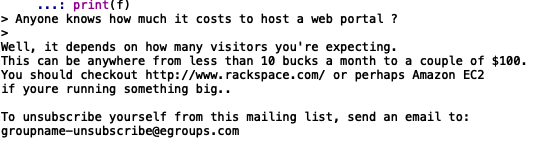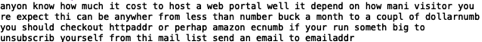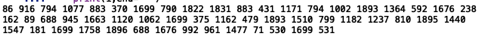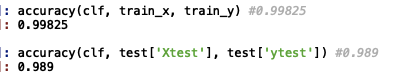15个权重最高的词，和作业中有些微差别